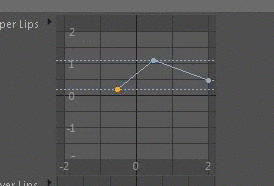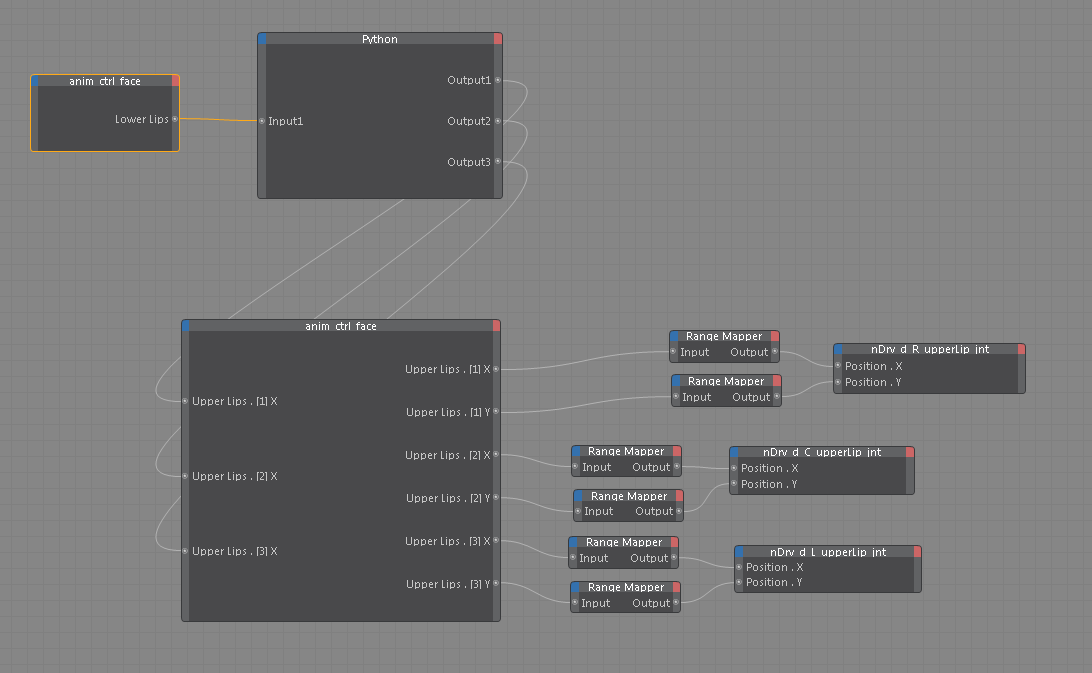# Create a custom datatype for userdata????

On 21/09/2015 at 08:55, xxxxxxxx wrote:

Hello.
Im wondering if its possible to create my own datatype for userdata with python.
I would like to create my own spline datatype if possible.

On 22/09/2015 at 02:20, xxxxxxxx wrote:

Hello,

it is only possible to create custom Datatypes or custom GUIs using the C++ SDK with CustomDataTypeClass and iCustomGui. See also you other thread "How to create a cutom data type for UserData? ".

Best wishes,
Sebastian

On 22/09/2015 at 04:22, xxxxxxxx wrote:

Hello.
Yes that my thread over at c4dcafe.

Im not that much into c++.

Since its not possible to create a custom data type with python.

Would it be possible to manipulate the Spline datatype in the userdata using python then?
As you saw in my other thread i want to restrict/clamp the points on the spline.

Basically i want.
clamp point 1's X axis to a range of -2 to 0.5, Y axis -2 to 2
clamp point 2's X axis to a range of -0.5 to 0.5, Y axis -2 to 2
clamp point 3's X axis to a range of 0.5 to 2, Y axis -2 to 2

Is this possible then ?

On 22/09/2015 at 08:29, xxxxxxxx wrote:

So after messing around a bit i see that there is a python node in the expresso editor.
i tried adding a python node to my expresso which drives the lip splines.

i can see that the python script reads the spline values fine when i change them.
however it will not clamp like i want to. is there somethign wrong with my SetKnot ?

``````
import c4d
from c4d import documents, utils, internals
LIP_UPPER_ID = 14 # upper lip spline

def main() :
obj = doc.GetActiveObject()
uls = obj[c4d.ID_USERDATA, LIP_UPPER_ID]
knots = uls.GetKnots()
if len(knots) == 3:
k1 = knots['vPos']
k2 = knots['vPos']
k3 = knots['vPos']
k1X = k1
k1Y = k1
if k1X < -0.5:
k1X = -0.5
uls.SetKnot(index = 0, vPos = c4d.Vector(k1X, k1Y, 0))
``````

On 22/09/2015 at 10:21, xxxxxxxx wrote:

Hello,

if I understand correctly you want to create individual constraints for each point? That is not possible with the existing Spline GUI.

Best wishes,
Sebastian

On 22/09/2015 at 11:04, xxxxxxxx wrote:

Yes thats exactly what i want to do.
I also refused to belive youAfter hours of messing around i finally got it to work.
seeing that the SetKnot way did not do anything.

By feeding the output of a Python node back into the obj "anim crtl face" i managed to clamp the values.
The 3 outputs are floats.

EDIT: the wrong spline is set in the input to the python node.
But this ibput isnt realy used so it doesnt matter.

This method is not perfect but does the job for me.The Python code i used:

``````
import c4d
from c4d import documents, utils, internals

LIP_UPPER_ID = 14 # upper lip spline
LIP_LOWER_ID = 15 # lower lip spline

def main() :
global Output1
global Output2
global Output3
obj = doc.GetActiveObject()
uls = obj[c4d.ID_USERDATA, LIP_UPPER_ID]
knots = uls.GetKnots()
if len(knots) == 3:
k1 = knots['vPos']
k2 = knots['vPos']
k3 = knots['vPos']

k1X = k1
k1Y = k1

k2X = k2
k2Y = k2

k3X = k3
k3Y = k3

print k1X, k1Y
if k1X > -0.51:
k1X = -0.51

if k2X < -0.49:
k2X = -0.49

if k2X > 0.49:
k2X = 0.49

if k3X < 0.51:
k3X = 0.51

Output1 = k1X
Output2 = k2X
Output3 = k3X
``````